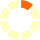# New York State Next Generation Learning Standards: 4.NBT.6

## Find whole-number quotients and remainders with up to four-digit dividends and one-digit divisors, using strategies based on place value, the properties of operations, and/or the relationship between multiplication and division. Illustrate and explain the calculation by using equations, rectangular arrays, and/or area models.Notes on and/or: Students should be taught to use strategies based on place value, the properties of operations, and the relationship between multiplication and division; however, when solving any problem, students can choose any strategy.Students should be taught to use equations, rectangular arrays, and area models; however, when illustrating and explaining any calculation, students can choose any strategy.